TUTORIALS
TUTORIALS HOME

GENERAL MATH
NUMBER SETS
ABSOLUTE VALUE & INEQUALITIES
SETS & INTERVALS
FRACTIONS
POLYNOMIALS
LINEAR EQUATIONS
GEOMETRY
FINITE SERIES
TRIGONOMETRY
EXPONENTS
LOGARITHMS
INDUCTION

CALCULUS
LIMITS
DERIVATIVES
RELATED RATES & OPTIMIZATION
CURVE SKETCHING
INTEGRALS
AREA & VOLUME
INVERSE FUNCTIONS

MAIN
HOME
TESTS
TUTORIALS
PROBLEMS
COMMON MISTAKES
STUDY TIPS
GLOSSARY
CALCULUS APPLICATIONS
MATH HUMOUR

### POLYNOMIALS TUTORIAL

A polynomial is a sum of one or more terms, where each term consists of a constant and one or more variables raised to some non-negative integer exponents. A polynomial with only one term is called a monomial, two terms, a binomial and three terms, a trinomial. Some examples of each type are listed below.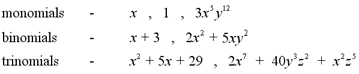The degree of an individual term in a polynomial is the sum of the exponents of the variables in that term. For example, the term x2y3 has a degree of 5, since the exponents of the variables x and y ( 2 and 3 respectively ) add up to 5.

Note : This is one of the laws of exponents, which will be covered in the exponents tutorial.

The degree of a polynomial is the largest degree of any of its individual terms. The polynomial x2y3 + xy3 + 5y would have a degree of 5, since that is the largest degree of each of its terms.

A root of a polynomial p(x) is a number, a, such that p(a)=0. If a is a root of a polynomial p(x), then p can be factored such that p(x)=(x-a)q(x) for some polynomial q(x). The different methods of factoring polynomials are found in the section below.

# Factoring Binomials

The simplest method of factoring binomials is to remove factors that are common to different terms. This method does not work in all situations. The formulas listed below can be used to factor binomials that fit the specific situation.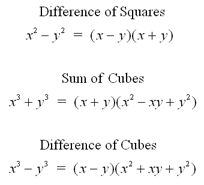# Examples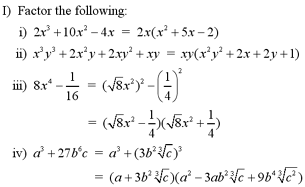# Factoring Trinomials

Quadratic trinomials have the form ax2 + bx + c. When factoring these trinomials, we want to write them as a product of two binomials. The two types of quadratic trinomials are listed below.

Monic quadratic trinomials are expressions where the leading coefficient (a) is equal to 1. For example, x2 + 7x + 12 is a monic quadratic trinomial. These trinomials are the simplest to factor. For the general monic quadratic trinomial, x2 + bx + c, we must find the roots of the polynomial, x1 and x2, such that x2 + bx + c = (x-x1)(x-x2). By expanding the factorization, we see that x2 + bx + c = x2 - (x1+x2)x + (x1x2). From this expansion, we get the formulas b = -(x1+x2) and c = x1x2. We can use these formulas to find the roots of the polynomial, if it can be factored.

The simplest way to factor monic quadratic trinomials is to look for integer roots. If we cannot find integer roots however, it does not mean that the trinomial can't be factored. We must use the quadratic formula, which is given in the quadratic equations tutorial, to find the non-integer roots of the quadratic trinomial. If no roots exist, however, then the trinomial cannot be factored.

# Examples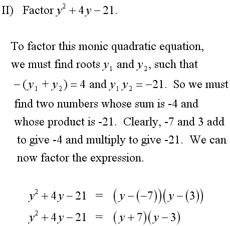Nonmonic quadratic trinomials are expressions where the leading coeffecient (a) is not equal to 1. For example 6x2 - 11x - 7 is a nonmonic quadratic trinomial. To factor these trinomials, we must again find roots x1 and x2 such that ax2+bx+c = a(x - x1)(x - x2).

The simplest way to factor a nonmonic trinomial is to find two integers m and n, such that m + n = b and m x n = ac. After finding the two integers, substitute the bx term for the sum of terms mx + nx. Then remove common factors. If no integers can be found, it does not necessarily mean that the polynomial cannot be factored. We must find the non-integer roots of the polynomial using the quadratic formula. If there are no roots of the polynomial, it cannot be factored.

# Examples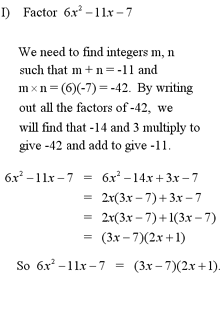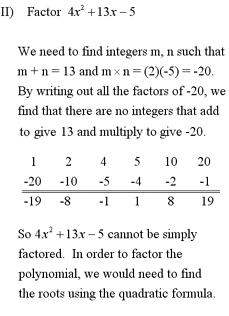Note: For an example of factoring a polynomial using grouping, see question #1 in the Additional Examples section at the bottom of the page.

# The Fundamental Theorem of Algebra

The Fundamental Theorem of Algebra states : Any polynomial with real coefficients can be split into the product of linear or quadratic factors.

However, although the linear or quadratic factors are polynomials, they may not be able to be split any further. For example, the quadratic equation y = x2 + x + 1 cannot be split any further.

Note: The complete Fundamental Theorem of Algebra contains references to complex numbers which is more complicated of a theorem than is required for the scope of the first year calculus course. The theorem in its place above is a simplified version. More information on the Fundamental Theorem of Algebra can be found in any first year algebra textbook.

# Rational Expressions

A rational expression is a quotient where the numerator and the denominator are both polynomials. The rules for operations of fractions can also be applied to operations involving rational expressions.

# Examples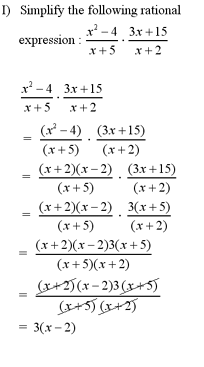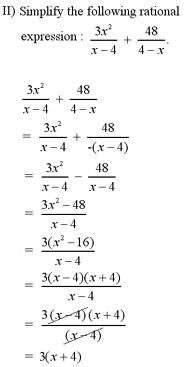Note: For a more complex example of performing arithmetic operations with rational expressions, see question #2 in the Additional Examples section below.

1 | Example of a polynomial factored using grouping
2 | Example of operations performed on rational expressions

| Top of Page |

COURSE HOMEPAGES
MATH 1036
MATH 1037

FACULTY HOMEPAGES
Alex Karassev
Ted Chase